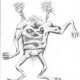Pathallea: The Basics of Thoul, part 2.

Other Pronoun Forms:

Where = larr            There = lirr               Here = lurr

When = yarr            Then = yirr                If = yurr                   Now = yorr

Whence = warr        Thence = wirr           Hence = wurr

Wherefor = sharr     Therefor = shirr        Herefor = shurr       How come = sherr

Which = theks         Whether = thoks

>>”Wherefor” = “Why”; “Herefor” = “How.”

Prepositions:

To = yil                     From = yel             Near = yilk            Away = yelk

In = il                        Out = el                  Inside = ilk           Outside = elk

On = ik                     Off = ek                  Upon = ic             Off of = ec

Up = ber                   Down = bor            Over = berk         Under = bork

Before = beri            After = bori             In front = berik     Behind = borik

Within = ilpha           Without = elpha      With = pha

Through = phik           Across = phuk          Along = phak         At = yeck

Beside = thik               Between = thuk       Among = thak        Beyond = yock

Conjunctions:

And = niks                Or = nuks               Either = uks

Neither = huks          Nor = muks            For = rok             Against = rek

And/or = meks          And not = miks

So = aks                   Yet = eks                But = oks

Because = gegek      For = ge                  Other = ora          Another = orra

Thus = thiks              Rather = thuks       Though = thaks    Than = theck    Same = thack

As = ock                    Like = eck        Unlike = meck           As () as = ok-()-k

All = a          Many = um            Some = om           Few = em            None = im

Always = am         Any = eh        Few = mum          Much = u        Every = mam

More = ner           Most = ser          Ever = ker     Never = iker       Even = kerk

Although = gedeg    Almost = gekeg      Altogether = gedek     Also = gek

Since = phiks         Thorough = phigik      Well = phuk     Again = phis        About = phus

>>The above prepositions, conjunctions, and adverbs are arranged according to word similarities; not so much according to their actual semantic value (although that is still mostly the case).  For example, ‘because’ is not an adverb, but conjugates like one (due to it deriving from ‘of’).

Numbers:

Thoul numbers are easily conjugated, and thus overall more on the boring side of things…

0 = ih                0th = ihkya                   (ihtya)                          11 = eshe

1 = e               1st = ekya                  Once = etya              12 = eshes

2 = es               2nd = eskya                 Twice = estya             13 = esher

3 = er                3rd = erkya                  Thrice = ertya             20 = esesh

4 = em              4th = emkya                 (emtya)                        30 = eresh

5 = ey               5th = eykya                  (eytya)                         100 = ash

6 = eye           6th = eyekya              (eyetya)                     1,000 = ath

7 = eyes           7th = eyeskya              (eyestya)                     1,000,000 = ay

8 = eyer            8th = eyerkya              (eyertya)

9 = eyem           9th = eyemkya             (eyemtya)

10 = esh            10th = eshkya              (eshtya)

Half = ecesa                         Three-quarter = ercema

Third = ecera                        Two-third = escera

Quarter = ecema                  Three-half = ercesa

Fifth = eceya                        Four-third = emcera

Sixth = eceyea

Seventh = eceyesa

Eighth = eceyera

Ninth = eceyema

Tenth = ecesha

AND THAT CONCLUDES PART TWO!  More on Thoul grammar to come, eventually…

2 thoughts on “Pathallea: The Basics of Thoul, part 2.”

1.oogenhand says:

Interesting; case affixes being prefixes. As in Esperanto, the regularity tends to make it hard to distinguish the “correlatives”. The other end of the equation is suppletion: “Sordäm?” = “Why?”, “Pelgut!” = “That’s why!”/”Therefore…”

Like

1.boteotu says:

Neat… Thanks!

Like